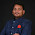# Design of Tension Members:

For this we need IS 800 - 2007
Tension members are linear member in which axial forces act to cause elongation. Such member can sustain load upto the ultimate load, which stage they may fail by rupture at a critical section.

The factored design tension (T) in members shall satisfy:
T < Td
where, Td is Design strength of member.

## #Td is classified under as for Bolted Connection:

1. Tdg
2. Tdn
3. Tdb
Consider Td value whichever is less.

### 1) Due To Yeild Strength (Tdg):

Cl 6.2 Page 32
Tdg = Ag.fy/rmo
where,
Ag=Gross Area of Cross Section
fy=Yeild Stress of Material

### 2) Due To Rupture of Critical Section (Tdn):

Cl 6.3 Page 32
Tdn = 0.9.An.fu/rml
where,
An=(b-n.dh)t   For Chain Bolting
fu=Ultimate Stress of Material

Cl 6.3.3
If there is Single Angle Section then,
Tdn =(.9Anc.fu/rml) + (beta.Ago.fy/rmo)
where,
beta=1.4-0.076(w/t)(fy/fu)(bs/Lc) < (fu.rmo/fy.rml)
>0.7
w=outstanding width
t=Thickness of Leg
bs=shear lag width
Lc=Length of end connection
An=Net area of Total Cross-section, as told above
Anc=Net Area of Connection Leg
Ago=Gross Area of the Outstanding Leg

### 3) Due to Block Shear (Tdb):

Cl 6.4
Tdn1 = (Avg.fy/ rmo.root3) + (0.9.Atn.fu/rml)
Tdn2 = (0.9.Avn.fu/rml.root3) + (Atg.fy/rmo)
where,
Avg = Lv.t
Avn= (Lv-n.dh).t
Atg = Lt.t
Atn = (Lt-ndh).t

## #Td for Welded Connection:

The Block Shear Strength Should be checked for welded end connection for member which can shear off as block.

For this Avg = Avn = Lv.t
and Atg = Atn = Lt.t
where,
Lv is Longitudinal Length
Lt  is Transverse Length

1.You have done a good job by publishing this article about tdg course online .These course are so helpful for get lots of formulas about tdg.I appreciate your efforts which you have put into this article, It is a beneficial article for us. Thanks for sharing such informative thoughts.tdg course online

1.Thank you so much for your comment, and will work on such articles do check my blog.